### Showing videos fromEddie Woo with a total of 4,051 videos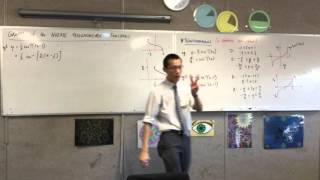08:47
Graphs of the Inverse Trig Functions (3 of 3: WARNING - Shifting and transforming the WRONG way)
3y1m ago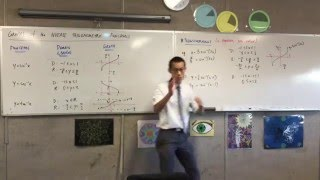08:45
Graphs of the Inverse Trig Functions (2 of 3: Transformations of the basic inverse trig functions)
3y1m ago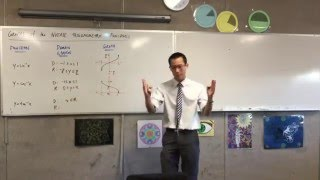09:20
Graphs of the Inverse Trig Functions (1 of 3: Reviewing the domain, range and shape)
3y1m ago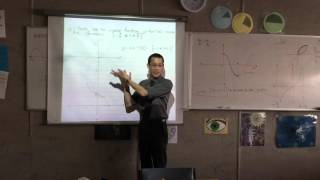04:09
Inverse Trigonometric Functions (4 of 4: Graphing the Shape of the inverse Trig Functions)
3y1m ago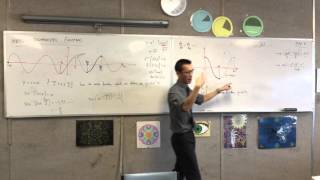07:32
Inverse Trigonometric Functions (3 of 4: Determining the domain of cos¯¹x and tan¯¹x)
3y1m ago08:02
Inverse Trigonometric Functions (2 of 4: Justifying the Choice of Domain)
3y1m ago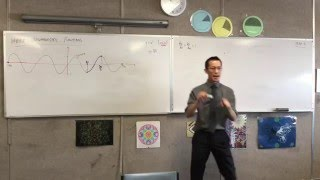08:11
Inverse Trigonometric Functions (1 of 4: Choosing a Domain for the Inverse of sine)
3y1m ago09:54
Properties of Inverse Functions (3 of 3: Relationship between inverse and original symmetry)
3y1m ago10:31
Properties of Inverse Functions (2 of 3: Relationship of derivatives of the original and inverse)
3y1m ago13:32
Properties of Inverse Functions (1 of 3: If f(x) is increasing, f¯¹(x) is also increasing)
3y1m ago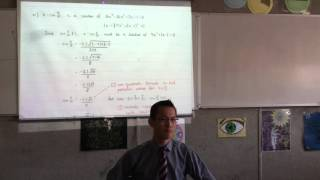05:17
Extension II Assessment Review (5 of 5: De Moivre's Theorem and Polynomials)
3y1m ago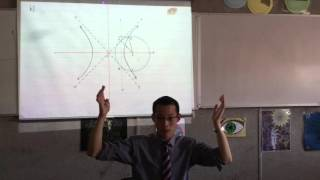09:59
Extension II Assessment Review (4 of 5: Polynomials and Conics Questions Explained)
3y1m ago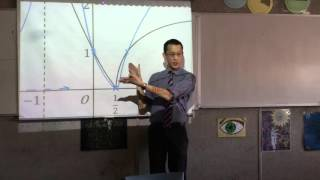04:47
Extension II Assessment Review (3 of 5: Raising Original Graphs to Powers & Reciprocating)
3y1m ago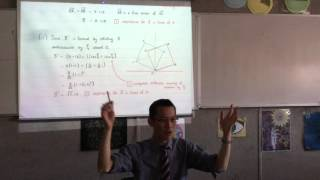05:39
Extension II Assessment Review (2 of 5: Complex Conjugate Root Theorem, DMT & Geometry)
3y1m ago10:38
Extension II Assessment Review (1 of 5: Multiple Choice Questions Section)
3y1m ago06:42
Inverse Functions (4 of 4: Restriction the Domain to get an Inverse Function of Even Functions)
3y1m ago07:24
Inverse Functions (3 of 4: What happens when you evaluate f(f^-1(x)))
3y1m ago06:29
Inverse Functions (2 of 4: Finding the Inverse and the geometrical properties of Inverse Function)
3y1m ago07:13
Inverse Functions (1 of 4: Outline of properties of inverse functions)
3y2m ago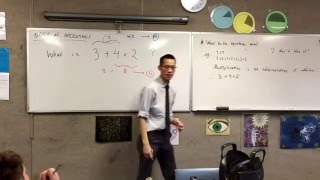10:49
Introduction to the Order of Operations (What comes first?)
3y2m ago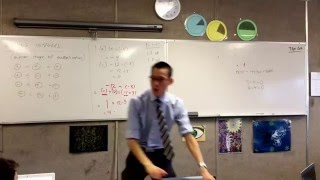08:25
Dividing Integers (Dealing with negative divisors and dividends)
3y2m ago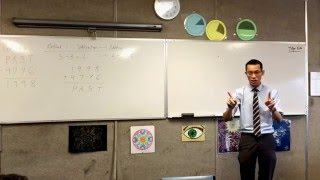10:24
Harder Integer Question (Adding and Subtracting Integers to find Unknown Integers)
3y2m ago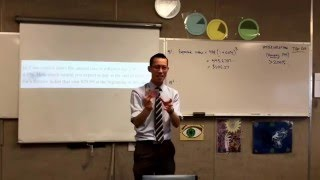07:56
Inflation (2 of 2: Some calculations related to inflation)
3y2m ago15:25
Inflation (1 of 2: Basic Outline of Inflation [and deflation] and the how it affects products)
3y2m ago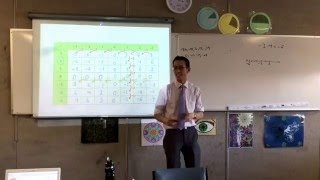05:40
Ascending and Descending Integers (3 of 3: Recognising the mathematical relation between cells)
3y2m ago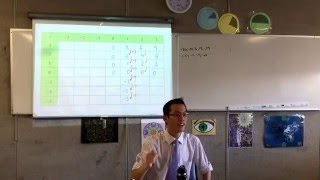08:50
Ascending and Descending Integers (2 of 3: Noticing patterns in multiplications)
3y2m ago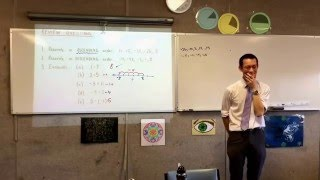05:43
Ascending and Descending Integers (Evaluating Ascending, Descending on Number lines)
3y2m ago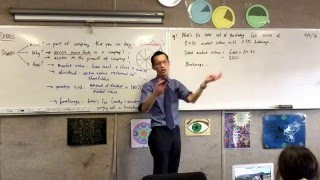07:30
Shares (2 of 2: Calculating the total amount required to buy a set number of shares)
3y2m ago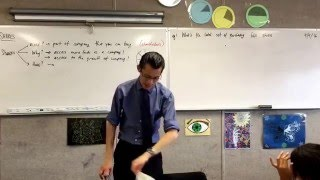09:49
Shares (1 of 2: Exploring Shares and surrounding terminology and jargon)
3y2m ago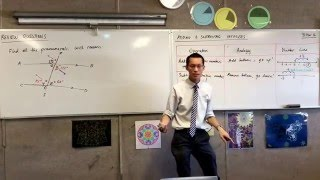11:58
Adding and Subtracting Integers (Applying operations to integers and visualising with Number line)
3y2m ago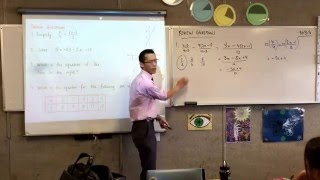11:00
Reviewing Fractions with Algebra and linear algebraic relations
3y2m ago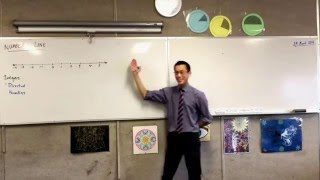06:21
The Number Line (How do Integers fit on a Number line?)
3y2m ago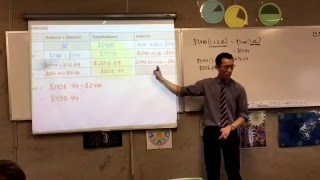03:56
Interest (3 of 3: Identifying and taking advantage of the patterns surrounding Compound Interest)
3y2m ago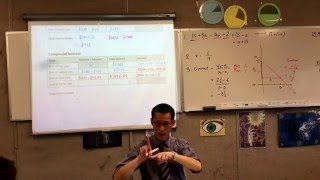05:38
Interest (2 of 3: Identifying difference between Compound and Simple interest )
3y2m ago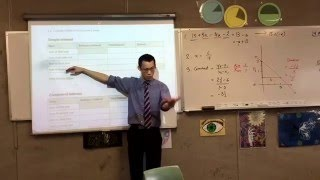08:52
Interest (1 of 3: Calculating amount of Simple Interest earned over 4 years)
3y2m ago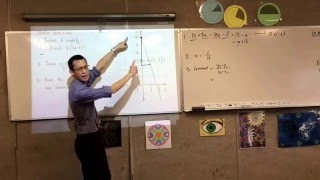08:17
Reviewing Expanding and Simplifying, Fractional algebra and Gradient
3y2m ago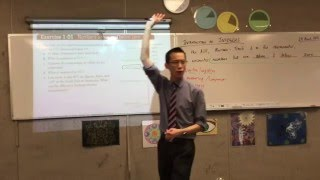08:16
Introduction to Integers (2 of 2: Solving problems with Integers)
3y2m ago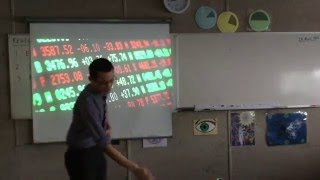08:02
Introduction to Integers (1 of 2: Linking Numbers that are above & below zero)
3y2m ago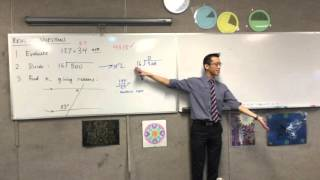05:07
Reviewing Multiplication, Division and Properties of Angles on Parallel Lines
3y2m ago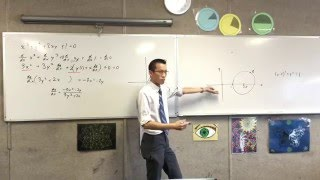08:04
Implicit Differentiation (Differentiating a function without needing to rearrange for x or y)
3y2m ago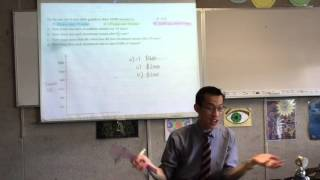12:55
Simple Interest (4 of 4: Using Information to identify a linear relationship to graph)
3y2m ago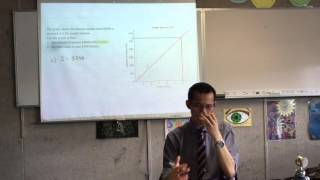04:26
Simple Interest (3 of 4: Using a graphical relationship to find information without calculations)
3y2m ago05:35
Simple Interest (2 of 4: Extrapolating Familiar Mathematical Notation from a question)
3y2m ago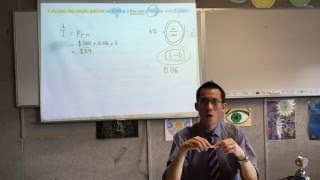09:57
Simple Interest (1 of 4: Relevance of AM1 and AM2 topics in Simple Interest)
3y2m ago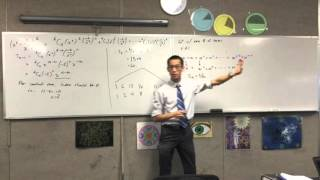08:54
Harder Series and Sequences (Finding the sum of the odd terms in a given series)
3y2m ago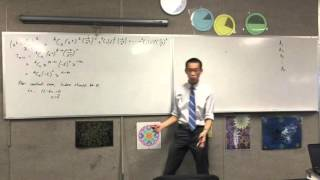10:46
Binomial Theorem (Finding a specified term using Commutations and a general Index Notation )
3y2m ago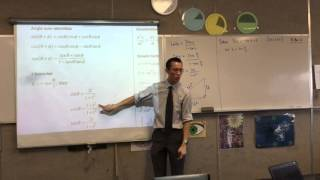09:55
"T" Results (Using t results to solve trigonometric equations)
3y2m ago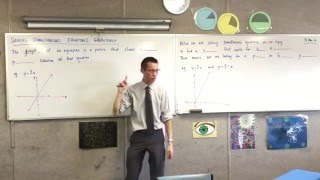07:26
Graphically Solving Simultaneous Equations (Solving by using a simple visual argument)
3y2m ago02:09
Solving Limits involving properties of Trigonometric Functions as they approach the origin.
3y2m ago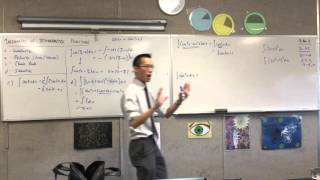07:45
Integration of Trigonometric Functions (3 of 3: Using Trig Identities to evaluate cos^2(x))
3y2m ago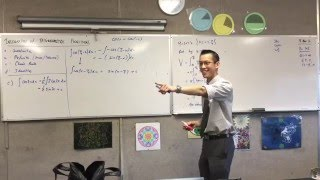06:26
Integration of Trigonometric Functions (2 of 3: Manipulating the function to simplify integral)
3y2m ago08:58
Integration of Trigonometric Functions (1 of 3: Introduction to uses and some examples)
3y2m ago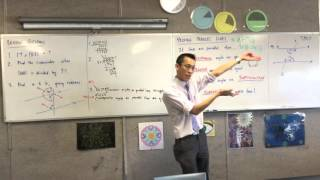10:15
Proving Parallel Lines (2 of 2: Using the properties of angles on parallel lines for proofs)
3y2m ago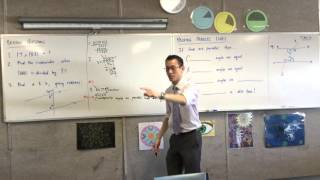04:37
Proving Parallel Lines (1 of 2: Reviewing Multiplication, Division and Parallel Line Angles)
3y2m ago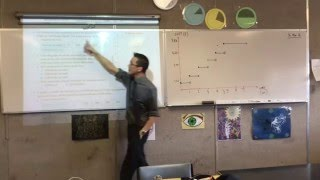04:27
Piecewise Graphs (3 of 3: Solving problems with a piecewise function)
3y2m ago07:48
Piecewise Graphs (2 of 3: Representing information graphically to visualise the trend)
3y2m ago10:49
Piecewise Graphs (1 of 3: Using Piecewise Graphs to represent data)
3y2m ago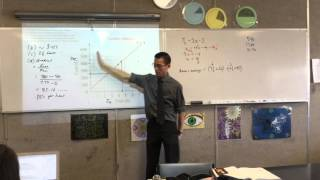09:37
Gradient of linear functions (Extrapolating the meaning behind the function plotted)
3y2m ago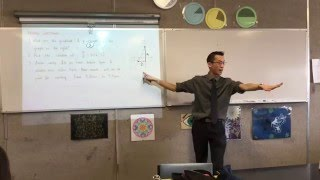06:36
Linear Equations (Reviewing gradient & solving linear equations)
3y2m ago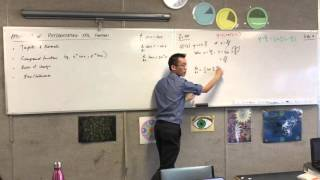07:55
Application of Differentiating Trig Functions (2 of 2: Tangents and normals of a trig function)
3y2m ago09:11
Applications of Differentiating Trig Functions (1 of 2: How is differentiating used in maths?)
3y2m ago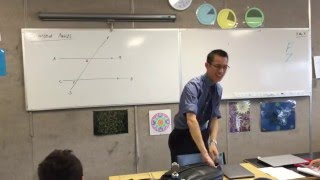12:35
Patterns on Parallel Lines (2 of 2: Cointerior Angles on Parallel Lines)
3y2m ago11:01
Patterns on Parallel Lines (1 of 2: Proving that Alternate Angles on Parallel Lines are equal)
3y2m ago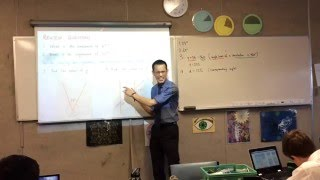06:00
Revolution Angles and Corresponding Angles (How to use mathematical reasoning to justify results)
3y2m ago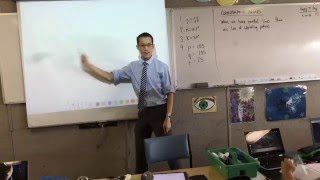11:48
Corresponding Angles (Finding patterns in angle side on parallel lines)
3y2m ago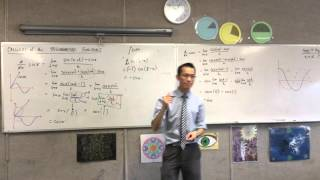06:17
Calculus of Trigonometric Functions (2 of 2: Using derivative of sinx and cos x to find d/dx(tanx))
3y2m ago12:36
Calculus of Trigonometric Functions (1 of 2: Using First Principles to find d/dx of sinx)
3y2m ago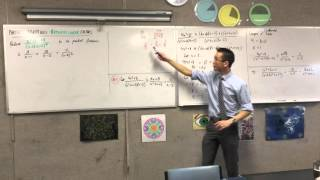09:41
Partial Fraction: Repeated Linear Factors (Technique for Breaking down into partial fractions)
3y2m ago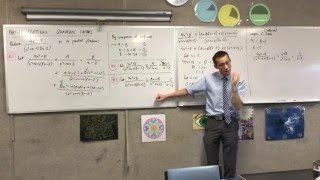06:37
Partial Fractions: Quadratic Factors (2 of 2: Problems with Quadratic Factors and Solution)
3y2m ago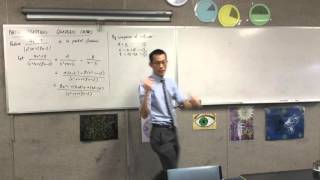10:45
Partial Fractions: Quadratic Factors (1 of 2: Issues with non-linear denominator factors)
3y2m ago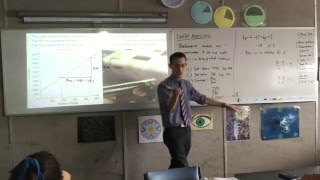08:46
Linear Modelling (3 of 3: Using the Gradient to find a relation between two variables)
3y2m ago